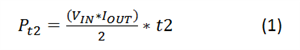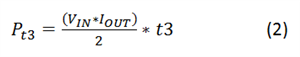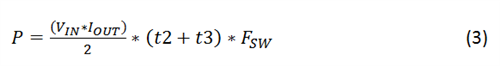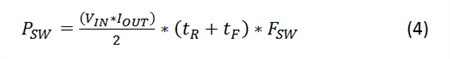# DC/DC converter datasheets – System losses demystified

Other Parts Discussed in Post: LM2673, LM43603

Welcome back to the DC/DC converter datasheets series. Whereas in the last installment I covered system efficiency, in this installment I will discuss DC/DC regulator component switching losses, starting with what was Figure 3 in part 1 (Figure 1 here): the image of the VDS and ID curve against time.Figure 1: Switching losses

Let’s first look at the switching losses in the integrated high-side MOSFET. At the beginning of every switching cycle, the driver starts supplying current to the gate of the integrated MOSFET. From part 1, you know that the MOSFET has parasitic capacitances across its terminals. During the first time period (t1 in Figure 1), the gate-source voltage (VGS) is approaching the MOSFET’s threshold voltage, VTH and the drain current is zero. The power loss during this period is therefore zero. In time period t2, the parasitic input capacitance (CISS) of the MOSFET begins to charge and the drain current starts flowing through the MOSFET, increasing linearly. For a buck topology, this current is the load current and the drain-source voltage (VDS) is the input voltage (VIN). Therefore, during the second time period (t2), there is a power loss that can be expressed as Equation 1:Once the input parasitic capacitor of the MOSFET is charged, the load current flows through the MOSFET and VDS starts to fall. This time is also called “Miller time” because this time is spent just charging the Miller capacitance (CGD). During Miller time the drain current is constant at IOUT and VDS is falling from VIN. The power loss during this time is expressed as Equation 2:Adding the total switching loss results in Equation 3:Notice that in Figure 1, t2 is considerably shorter than the third time period (t3). Therefore in the equations, you could estimate the loss as just those in the time period t3. These finite transition times occur twice in a period: once when the MOSFET turns on (charging the parasitic capacitance) and once when it turns off (discharging the parasitic capacitance). Therefore, estimating the time t3 in both cases as the rise and fall times of the MOSFET, you can estimate the switching loss using Equation 4:The switching loss is frequency- and input voltage-dependent. Therefore, at higher input voltages and switching frequencies, the overall efficiency is comparatively reduced. At light loads, the LM2673 nonsynchronous buck regulator enters discontinuous conduction mode. In this mode, the device still maintains the switching frequency. With every cycle, power is still dissipating inside the integrated circuit (IC). Therefore, even though conduction loss is not a factor at light loads, due to the always-present switching loss, device efficiency is affected. And since the average power delivered to the output is very low, the overall efficiency of the device is also low.

Newer regulators from the SIMPLE SWITCHER® family now come equipped with pulsed frequency modulation (PFM) that lowers the switching frequency when the load is reduced. This enables the regulator to maintain high efficiency all the way down to very light or no loads.

Because the LM2673 is a nonsynchronous device, it has a catch diode that gets forward-biased when the MOSFET turns off. The same principle of a non-zero transition time applies to the catch diode as well. But the voltage swing of the diode is just from ground to the forward voltage drop (VF) of the diode, making the switching losses in the diode negligible. You can also ignore switching losses occurring in the inductor’s core because SIMPLE SWITCHER regulators like the LM2673 have a comparatively lower switching frequency of 250 kHz.

In the next (and final) installment in this series, I will discuss the conduction losses in the MOSFET and passive components of the DC/DC regulator circuit, and present an expression for total losses and resulting efficiency.